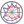Course title
Mathematical Programming
 YAMAZAWA HiroshiHIROSE SampeiCourse description
Mathematical programming is a method for maximizing (minimizing) an objective function under constraints. The problems handled by this method are also called mathematical programming problems or optimization problems, and appear very often as mathematical models of engineering problems. For example, a production planning problem to produce a product with limited resources and maximize profit, or a transportation problem to minimize the cost of transporting a product from multiple factories to a delivery destination.
In this class, students learn how to solve basic linear and nonlinear programming problems. In addition, students will learn how to perform calculations using Excel.
Purpose of class
The purpose of this class is to understand the purpose of mathematical programming and to be able to calculate not only by hand but also by using a computer.
Goals and objectives
1. To be able to solve linear programming problems.
2. To be able to solve nonlinear programming problems.
3. To be able to solve mathematical programming problems using Excel.
Language
Japanese
Class schedule

Class schedule HW assignments (Including preparation and review of the class.) Amount of Time Required
1. Introduction to mathematical programming problems, typical linear programming problems, and graphical solution methods Read through the handouts and the textbook p.1-p.6, p.23-p.41 and solve the problems in the handouts before class. After class, review and solve the questions and examples. 190minutes
2. Simplex method 1 Read through the handouts and the textbook p.41-p.53 and solve the problems in the handouts before class. After class, review and solve the questions and examples. 190minutes
3. Simplex method 2 Read through the handouts and the textbook p.41-p.53 and solve the problems in the handouts before class. After class, review and solve the questions and examples. 190minutes
4. Two stage simplex method Read through the handouts and the textbook p.54-p.66 and solve the problems in the handouts before class. After class, review and solve the questions and examples. 190minutes
5. Duality and Sensitivity Analysis Read through the handouts and the textbook p.83-p.98 and solve the problems in the handouts before class. After class, review and solve the questions and examples. 190minutes
6. Lagrange's Method of Undetermined Multipliers Read through the handouts and solve the problems in the handouts before class. After class, review and solve the questions and examples. 190minutes
7. Karush-Kuhn-Tucker Condition Read through the handouts and the textbook p.127-p.139 and solve the problems in the handouts before class. After class, review and solve the questions and examples. 190minutes
8. Descent method Read through the handouts and the textbook p.139-p.144 and solve the problems in the handouts before class. After class, review and solve the questions and examples. 190minutes
9. Examination and its explanation Preparation of examination. 190minutes
10. Excel Basics (Functions and Logical Functions) Read through the handouts, study by video, and solve practice problems on the handouts before class. 190minutes
11. Linear and nonlinear problems with Excel (using solvers) Read through the handouts and the textbook p.66-p.82 and solve the problems in the handouts before class. After class, review and solve the questions and examples. 190minutes
12. Solving 1- and 2-variable descent methods with Excel Read through the handouts, study by video, and solve practice problems on the handouts before class. 190minutes
13. Descent Method Solution with VBA Read through the handouts, study by video, and solve practice problems on the handouts before class. 190minutes
14. Examination and its explanation Preparation of examination. 190minutes
Total. - - 2660minutes
Relationship between 'Goals and Objectives' and 'Course Outcomes'

Reports and little examinations Examination 1 Examination 2 Total.
1. 10% 15% 25%
2. 10% 15% 25%
3. 50% 50%
Total. 20% 30% 50% -
Evaluation method and criteria
Examinations and reports will be examples, exercises, and questions of the same level as those in the handouts or textbook.
Examination 1 (45%), examination 2 (15%), reports (40%) will be converted into 100 points, of which 60 points or more will be considered passing.
Textbooks and reference materials
Textbook: わかりやすい数理計画法、坂和正敏、矢野均、西崎一郎、森北出版
Prerequisites
Review Differential and Integral Calculus 1, Linear Algebra 1, and Differential and Integral Calculus 2
Office hours and How to contact professors for questions
• Email for office hours and questions
Regionally-oriented
Non-regionally-oriented course
Development of social and professional independence
• Course that cultivates an ability for utilizing knowledge
Active-learning course
More than one class is interactive
Course by professor with work experience
Work experience Work experience and relevance to the course content if applicable
N/A 該当しない
Education related SDGs:the Sustainable Development Goals• 4.QUALITY EDUCATION